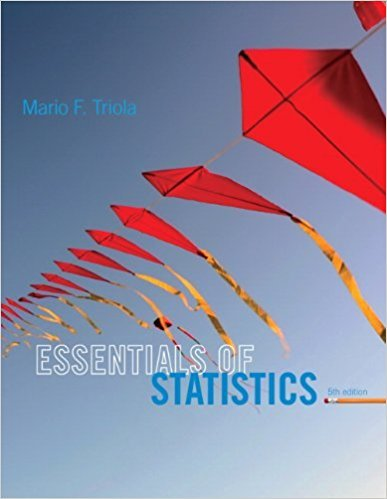×
Get Full Access to Statistics - Textbook Survival Guide
Get Full Access to Statistics - Textbook Survival Guide

×

Textbooks / Statistics / Essentials of Statistics 5

# Essentials of Statistics 5th Edition - Solutions by Chapter## Full solutions for Essentials of Statistics | 5th Edition

ISBN: 9780321924599Essentials of Statistics | 5th Edition - Solutions by Chapter

Solutions by Chapter
4 5 0 408 Reviews
##### ISBN: 9780321924599

The full step-by-step solution to problem in Essentials of Statistics were answered by , our top Statistics solution expert on 01/12/18, 03:16PM. Essentials of Statistics was written by and is associated to the ISBN: 9780321924599. This textbook survival guide was created for the textbook: Essentials of Statistics, edition: 5. This expansive textbook survival guide covers the following chapters: 50. Since problems from 50 chapters in Essentials of Statistics have been answered, more than 71049 students have viewed full step-by-step answer.

Key Statistics Terms and definitions covered in this textbook
• 2 k p - factorial experiment

A fractional factorial experiment with k factors tested in a 2 ? p fraction with all factors tested at only two levels (settings) each

• Adjusted R 2

A variation of the R 2 statistic that compensates for the number of parameters in a regression model. Essentially, the adjustment is a penalty for increasing the number of parameters in the model. Alias. In a fractional factorial experiment when certain factor effects cannot be estimated uniquely, they are said to be aliased.

• Alias

In a fractional factorial experiment when certain factor effects cannot be estimated uniquely, they are said to be aliased.

• Analytic study

A study in which a sample from a population is used to make inference to a future population. Stability needs to be assumed. See Enumerative study

• Arithmetic mean

The arithmetic mean of a set of numbers x1 , x2 ,…, xn is their sum divided by the number of observations, or ( / )1 1 n xi t n ? = . The arithmetic mean is usually denoted by x , and is often called the average

• Combination.

A subset selected without replacement from a set used to determine the number of outcomes in events and sample spaces.

• Comparative experiment

An experiment in which the treatments (experimental conditions) that are to be studied are included in the experiment. The data from the experiment are used to evaluate the treatments.

• Conditional probability

The probability of an event given that the random experiment produces an outcome in another event.

• Conidence level

Another term for the conidence coeficient.

• Consistent estimator

An estimator that converges in probability to the true value of the estimated parameter as the sample size increases.

• Curvilinear regression

An expression sometimes used for nonlinear regression models or polynomial regression models.

• Defect concentration diagram

A quality tool that graphically shows the location of defects on a part or in a process.

• Error propagation

An analysis of how the variance of the random variable that represents that output of a system depends on the variances of the inputs. A formula exists when the output is a linear function of the inputs and the formula is simpliied if the inputs are assumed to be independent.

• Error sum of squares

In analysis of variance, this is the portion of total variability that is due to the random component in the data. It is usually based on replication of observations at certain treatment combinations in the experiment. It is sometimes called the residual sum of squares, although this is really a better term to use only when the sum of squares is based on the remnants of a model-itting process and not on replication.

• Extra sum of squares method

A method used in regression analysis to conduct a hypothesis test for the additional contribution of one or more variables to a model.

• False alarm

A signal from a control chart when no assignable causes are present

• First-order model

A model that contains only irstorder terms. For example, the irst-order response surface model in two variables is y xx = + ?? ? ? 0 11 2 2 + + . A irst-order model is also called a main effects model

• Fisher’s least signiicant difference (LSD) method

A series of pair-wise hypothesis tests of treatment means in an experiment to determine which means differ.

• Fractional factorial experiment

A type of factorial experiment in which not all possible treatment combinations are run. This is usually done to reduce the size of an experiment with several factors.

• Generator

Effects in a fractional factorial experiment that are used to construct the experimental tests used in the experiment. The generators also deine the aliases.